Checkout JEE MAINS 2022 Question Paper Analysis : Checkout JEE MAINS 2022 Question Paper Analysis :

# Equation Of A Line In Three Dimensions

Equation of a line is defined as y= mx+c, where c is the y-intercept and m is the slope. Vectors can be defined as a quantity possessing both direction and magnitude. Position vectors simply denote the position or location of a point in the three-dimensional Cartesian system with respect to a reference origin. Further, we shall study in detail about vectors and Cartesian equation of a line in three dimensions. It is known that we can uniquely determine a line if:

• It passes through a particular point in a specific direction, or
• It passes through two unique points

Let us study each case separately and try to determine the equation of a line in both the given cases.

## Equation of a Line passing through a point and parallel to a vector

Let us consider that the position vector of the given point be

$$\begin{array}{l}\vec{a} \end{array}$$
with respect to the origin. The line passing through point A is given by l and it is parallel to the vector
$$\begin{array}{l}\vec{k} \end{array}$$
as shown below. Let us choose any random point R on the line l and its position vector with respect to origin of the rectangular co-ordinate system is given by
$$\begin{array}{l}\vec{r} \end{array}$$
.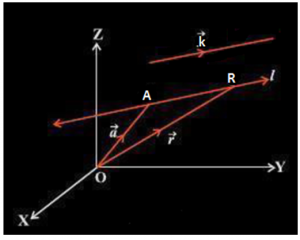Since the line segment,

$$\begin{array}{l}\overline{AR} \end{array}$$
is parallel to vector
$$\begin{array}{l}\vec{k} \end{array}$$
, therefore for any real number α,

$$\begin{array}{l}\overline{AR} \end{array}$$
= α
$$\begin{array}{l}\vec{k} \end{array}$$

Also,

$$\begin{array}{l}\overline{AR} \end{array}$$
=
$$\begin{array}{l}\overline{OR} \end{array}$$
–
$$\begin{array}{l}\overline{OA} \end{array}$$

Therefore, α

$$\begin{array}{l}\vec{r} \end{array}$$
=
$$\begin{array}{l}\vec{r} \end{array}$$
$$\begin{array}{l}\vec{a} \end{array}$$

From the above equation it can be seen that for different values of α, the above equations give the position of any arbitrary point R lying on the line passing through point A and parallel to vector k. Therefore, the vector equation of a line passing through a given point and parallel to a given vector is given by:

$$\begin{array}{l}\vec{r} \end{array}$$
=
$$\begin{array}{l}\vec{a} \end{array}$$
+ α
$$\begin{array}{l}\vec{k} \end{array}$$

If the three-dimensional co-ordinates of the point ‘A’ are given as (x1, y1, z1) and the direction cosines of this point is given as a, b, c then considering the rectangular co-ordinates of point R as (x, y, z):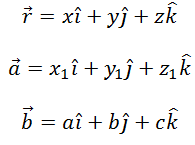Substituting these values in the vector equation of a line passing through a given point and parallel to a given vector and equating the coefficients of unit vectors i, j and k, we have,

<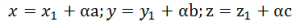Eliminating α we have: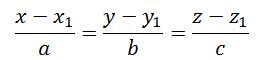This gives us the Cartesian equation of line.

### Equation of a Line passing through two given points

Let us consider that the position vector of the two given points A and B be

$$\begin{array}{l}\vec{a} \end{array}$$
and
$$\begin{array}{l}\vec{b} \end{array}$$
with respect to the origin. Let us choose any random point R on the line and its position vector with respect to origin of the rectangular co-ordinate system is given by
$$\begin{array}{l}\vec{r} \end{array}$$
.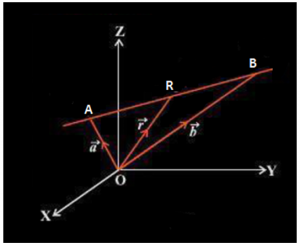Point R lies on the line AB if and only if the vectors

$$\begin{array}{l}\overline{AR} \end{array}$$
and
$$\begin{array}{l}\overline{AB} \end{array}$$
are collinear. Also,

$$\begin{array}{l}\overline{AR} \end{array}$$
$$\begin{array}{l}\vec{r} \end{array}$$
$$\begin{array}{l}\vec{a}\end{array}$$

$$\begin{array}{l}\overline{AB} \end{array}$$
$$\begin{array}{l}\vec{b} \end{array}$$
$$\begin{array}{l}\vec{a}\end{array}$$

Thus R lies on AB only if;

$$\begin{array}{l}\vec{r} – \vec{a} = \alpha (\vec{b} – \vec{a})\end{array}$$

Here α is any real number.
From the above equation it can be seen that for different values of α, the above equation gives the position of any arbitrary point R lying on the line passing through point A and B. Therefore, the vector equation of a line passing through two given points is given by:

$$\begin{array}{l}\vec{r} = \vec{a} + \alpha (\vec{b} – \vec{a})\end{array}$$

If the three-dimensional coordinates of the points A and B are given as (x1, y1, z1) and (x2, y2, z2) then considering the rectangular co-ordinates of point R as (x, y, z)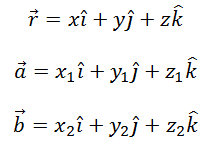Substituting these values in the vector equation of a line passing through two given points and equating the coefficients of unit vectors i, j and k, we have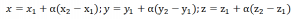Eliminating α we have: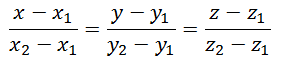This gives us the Cartesian equation of a line.